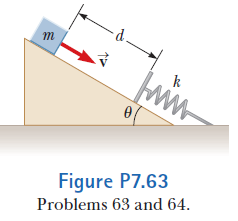# Problem: An inclined plane of angle θ has a spring of force constant k fastened securely at the bottom so that the spring is parallel to the surface. A block of mass m is placed on the plane at a distance d from the spring. From this position, the block is projected downward toward the spring with speed v as shown in Figure P7.63. By what distance is the spring compressed when the block momentarily comes to rest?

⚠️Our tutors found the solution shown to be helpful for the problem you're searching for. We don't have the exact solution yet.

###### Problem Details

An inclined plane of angle θ has a spring of force constant k fastened securely at the bottom so that the spring is parallel to the surface. A block of mass m is placed on the plane at a distance d from the spring. From this position, the block is projected downward toward the spring with speed v as shown in Figure P7.63. By what distance is the spring compressed when the block momentarily comes to rest?